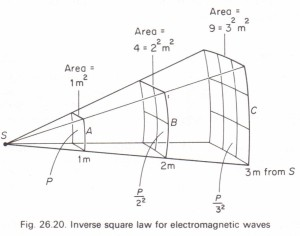# The inverse square law for electromagnetic waves

The inverse square law for electromagnetic waves

Let us suppose that an area of 1 m- which forms part of the surface of a sphere of radius I m receives wave energy from a point source placed at the centre S (Fig. 26.20).The inverse square law for electromagnetic waves

If P is the energy passing through this unit area in joules per second, i.e., if P is the power in watts, then we say that P is the wave intensity in W/m2.
If the distance is increased to 2 m it is clear from the geometry of the figure that the same power is now spread over an area of 22 = 4 m-. Consequently the wave intensity is reduced to ~ . Similarly, when the distance is increased to 3 m the wave. Wave intensity is defined as the power transmitted per unit area of the wavefront. In general, the wave intensity at any distance x from a point source is given by p X2 This relationship between wave intensity and distance is expressed by the inverse square law. The electromagnetic wave intensity from a point source in free space is inversely proportional to the square of the distance from the source.

Light as a special case. The measurement of light wave energy (photometry) is complicated by the fact that light sources contain non-visible radiation as well, e.g., ultraviolet and infrared. Special units called lux (not W1m2) therefore have to be used, but all the same, the inverse square law still holds. In this book we shall not be concerned with photometry.

Share This